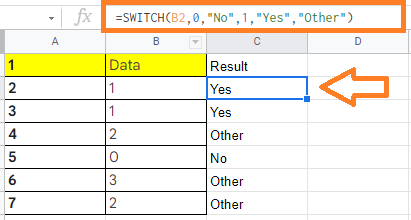# How to Use SWITCH function in Google Sheets? (With Examples)

Switch Function in Google Sheets: When an expression is tested against a list of cases, the Switch function returns the value of the first matching case, with an optional default value if no other conditions are matched. The Switch function also comes in handy, since it is very easy to read and understand even if we use multiple conditions. Let us understand how to use the Switch function with the help of Google Sheet tips provided on this page.

## SWITCH Function Syntax in Google Sheets

The syntax for using SWITCH function in Google Sheets are given below:

=SWITCH(expression, case1, value1, [case2, value2, …], [default])

• Expression: This specifies the values that the function will examine. This can be a specific cell reference, such as “B2, C2, A2 and so on.”
• Cases: The function looks for an exact match by comparing the value of a case to the expression.
• Values: This is what the function returns in the cell if the expression and the corresponding case are exact matches. The text “No” provides a good example of a return value.
• Default: This is optional. If none of the cases match the expression, an optional value will be returned as the last parameter.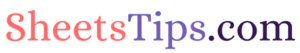### Using SWITCH Function with Two Conditions

Let us create the SWITCH function using the topmost row as a starting point. We are looking at row 2 in this example dataset.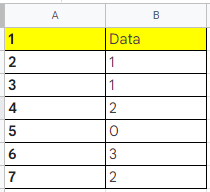Make a list of the case/value pairs. The first case/value pair in this example is 0 and “No,” whereas the second case/value pair is 1 and “Yes.”

So, our SWITCH function formula will be =SWITCH(B2,0,”No”,1,”Yes”)

Now consider the following dataset and follow the steps listed below to use the SWITCH function:

• Step 1: Move to cell C2.
• Step 2: Now use the formula =SWITCH(B2,0,”No”,1,”Yes”).
• Step 3: Press the “Enter” button.
• Step 4: Now drag the fill handle to other cells and you will see the results.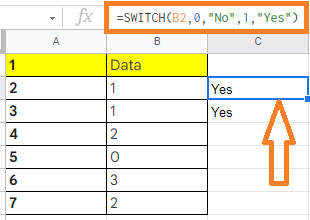### Using SWITCH Function with One Condition and 1 Default in Google Sheets

A SWITCH function will return an error if you don’t account for all possible case matches.

Fortunately, you can add the optional “default” value (also known as a fall-back value) to the end of the statement to return a value if no matching case is found.

If we’re using the SWITCH function to determine a student’s pass/fail status based on grades, we may set it to return “Fail” when an “F” case is detected, and “Pass” in all other cases.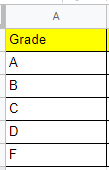So, our SWITCH function, in this case, will be =SWITCH(A2,”F”,”Fail”,”Pass”)

Now follow the steps as listed below to use the SWITCH Statement with default value:

• Step 1: Move to the row where you would like to use the SWITCH function. In this example, we are using the 2nd row, cell C2.
• Step 2: Now enter the formula as =SWITCH(A2,”F”,”Fail”,”Pass”).
• Step 3: Press the “Enter” button. You will see the results.
• Step 4: Now drag the formula applied cell to other rows and you will see the results.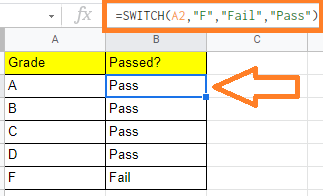### Using SWITCH Function with Multiple Condition and 1 Default in Google Sheets

Let us look at how to use the SWITCH function with several case/value pairs and a default option one more time. For that, we will use the same example dataset which we have used previously.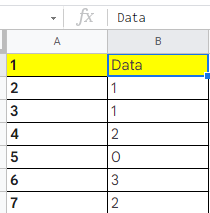For the above-pictured dataset, follow the steps listed below to use Switch Function:

• Step 1: Start building the SWITCH function in Google Sheets. For that move to cell A2. So our SWITCH function conditions are:
• If data contains “0”, then it should return “No“.
• If data contains “1”, then it should return “Yes“.
• If it does not contain both 0 and 1, then it should return Other.
• Step 2: So our SWITCH function formula will be =SWITCH(B2,0,”No”,1,”Yes”,”Other”). Enter the same formula in Cell B2.
• Step 3: Press the “Enter” button.
• Step 4: You will see the results. Now drag the formula applied cell to other rows to apply the SWITCH function and see the results.